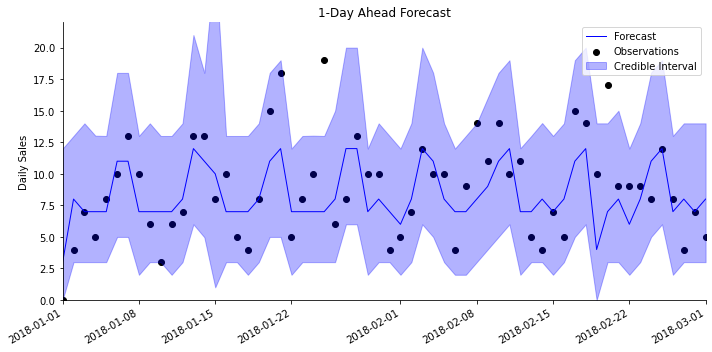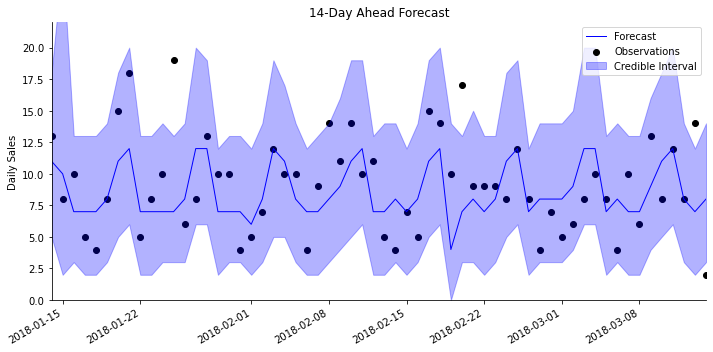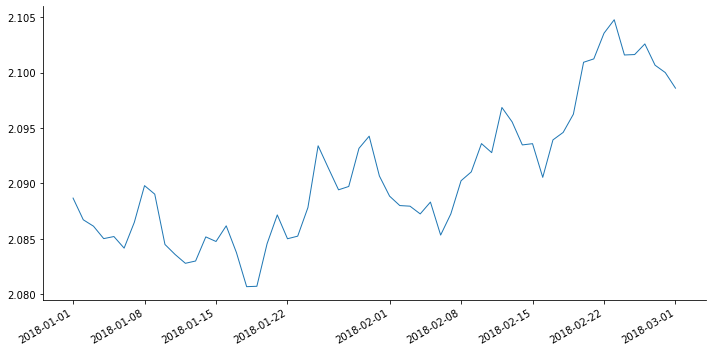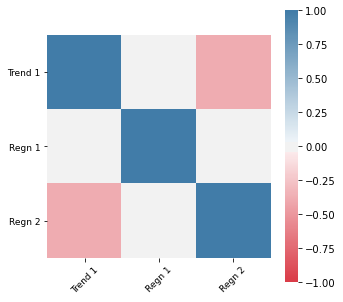Functions to help plot sequential forecasts and coefficients created during an analysis.

#### ax_style[source]

ax_style(ax, ylim=None, xlim=None, xlabel=None, ylabel=None, title=None, legend=None, legend_inside_plot=True, topborder=False, rightborder=False, **kwargs)

A helper function to define many elements of axis style at once.

#### plot_data_forecast[source]

plot_data_forecast(fig, ax, y, f, samples, dates, linewidth=1, linecolor='b', credible_interval=95, **kwargs)

Plot observations along with sequential forecasts and credible intervals.

The most commonly used plotting function, plot_data_forecast enables the plotting of sequential forecasts following an analysis. ax_style is a useful wrapper around some of the most common matplotlib.pyplot axis functionality.

A simple demonstration is given below. We start by running an analysis of simulated retail sales data:

from pybats.shared import load_sales_example2
from pybats.analysis import analysis
from pybats.plot import plot_data_forecast
from pybats.point_forecast import median
from pybats.loss_functions import MAPE

import matplotlib.pyplot as plt
import pandas as pd
from pandas.tseries.holiday import USFederalHolidayCalendar

prior_length = 21   # Number of days of data used to set prior
k = 14               # Forecast horizon
rho = 0.6           # Random effect discount factor to increase variance of forecast distribution
forecast_samps = 2000  # Number of forecast samples to draw
forecast_start = pd.to_datetime('2018-01-01') # Date to start forecasting
forecast_end = pd.to_datetime('2018-03-01')   # Date to stop forecasting

mod, samples, model_coef = analysis(data.Sales.values, data[['Price', 'Promotion']].values,
k, forecast_start, forecast_end, nsamps=2000,
family='poisson',
seasPeriods=, seasHarmComponents=[[1,2,3]],
prior_length=prior_length, dates=data.index, holidays=USFederalHolidayCalendar.rules,
rho=rho,
ret = ['model', 'forecast', 'model_coef'])

beginning forecasting


Next, we will plot the forecasts, both $1-$ and $14-$days ahead.

forecast = median(samples)

# Plot the 1-day ahead forecast
h = 1
start = forecast_start + pd.DateOffset(h - 1)
end = forecast_end + pd.DateOffset(h - 1)

data_1step = data.loc[start:end]
samples_1step = samples[:,:,h-1]
fig, ax = plt.subplots(figsize=(10,5))
plot_data_forecast(fig, ax,
data_1step.Sales,
median(samples_1step),
samples_1step,
data_1step.index,
credible_interval=95)

ax_style(ax, title='1-Day Ahead Forecast', ylabel='Daily Sales', ylim=[0,22])

passThe $1-$day ahead forecasts are generally quite accurate! It appears that most points are falling within the $95\%$ credible intervals. There is also a clear weekly pattern to the sales that the model has detected.

Let's take a look at the $14-$day ahead forecasts:

h = 14
start = forecast_start + pd.DateOffset(h - 1)
end = forecast_end + pd.DateOffset(h - 1)

data_14step = data.loc[start:end]
samples_14step = samples[:,:,h-1]
fig, ax = plt.subplots(figsize=(10,5))
plot_data_forecast(fig, ax,
data_14step.Sales,
median(samples_14step),
samples_14step,
data_14step.index,
credible_interval=95)

ax_style(ax, title='14-Day Ahead Forecast', ylabel='Daily Sales', ylim=[0,22])

passThese longer term forecasts are nearly as accurate as the $1-$day ahead forecasts. Note that the dates here start at January 13th, 14 days after the forecasting window began on January 1st.

#### plot_coef[source]

plot_coef(fig, ax, coef, dates, linewidth=1, linecolor=None, legend_inside_plot=True, coef_samples=None, **kwargs)

Plot coefficients over time.

This function is useful for plotting model coefficients over time. There is a detailed explanation of plotting the day-of-week seasonality coefficients in the sales forecasting example.

For this example, let's focus on any trends in the baseline level of sales. To track this, we will plot the intercept, which is the first coefficient in the trend term. The model coefficients have already been saved from the analysis we ran above.

plot_data = pd.DataFrame({'Intercept_Mean':model_coef['m'][:, mod.itrend]}, index=data.index)
plot_data = plot_data.loc[forecast_start:forecast_end]

fig, ax = plt.subplots(1,1, figsize=(10,5))
plot_coef(fig, ax, plot_data.Intercept_Mean, plot_data.index);It appears that the baseline level of sales are climbing throughout the forecast period!

#### plot_corr[source]

plot_corr(fig, ax, corr, labels=None)

Plot a correlation matrix with a heatmap.

This function is convenient for plotting a correlation matrix. It is commonly used to plot the correlations among model coefficients. It's a wrapper around the seaborn function sns.heatmap, with the colors scaled appropriately to highlight correlations.

To demonstrate, we'll plot the correlations among the trend and regression coefficients in the model from the examples above.

p = mod.ntrend + mod.nregn_exhol

cov = mod.R[:p,:p]
D = np.sqrt(cov.diagonal()).reshape(-1,1)
corr = cov/D/D.T

fig, ax = plt.subplots(figsize=(5,5))
plot_corr(fig, ax, corr = corr, labels=['Trend 1', 'Regn 1', 'Regn 2']);